# Primary Reformer Mechanical Design Assignment Help

In this section a mechanical design for the primary reformer tubes inside the furnace will be carried out. From the chemical design section we assumed each tube is 9 m long and has an internal diameter of 0.12 m.§ Design Pressure:

The pressure inside the vessels is 30 bar. 10% above operating pressure was considered for safety:

= (30-1) x 1.1

=31.9

=32 bar

= 3.19 N/mm2

§ Design Temperature:

Design temperature = 800oC (1073 K.)

§ Thickness of tube:

The minimum thickness required to resist internal pressure is :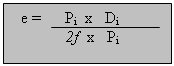## Primary Reformer Mechanical Design Assignment Help By Online Tutoring and Guided Sessions from AssignmentHelp.Net

e = the minimum thickness required.(mm)

Pi= Internal pressure. (kN/mm2)

Di=Internal diameter. (mm)

f= Design stress factor.

e = (120x3.19)/((2x115)-3.19)

e= 1.7 mm

We add 1mm for corrosion e= 1.7+1 =2.7 mm.

The 2 ends of each tube are designed to be closed by a head. There are three types of heads:

Ø For a standard Torispherical head:

§ Crown radius Rc = Di = 0.12m.

§ Knuckle radius= 6% of Rk =7.2x10-3m.

§ A head of this size would be formed by pressing no sides, therefore j= 1.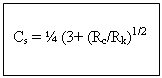Cs = Stress concentration factor for Torispherical heads.

Cs = ¼ (3 + ((0.12/7.2-3))-1/2)

Cs= 1.77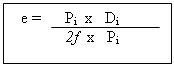e = (3.2 x 120 x 1.77)/ ((2x115) + 3.2 (1.77-0.2))

Thickness of head e = 2.9 mm.

Ø For a standard ellipsoidal head:Most standard ellipsoidal heads are manufactured with a major and minor axis ratio of 2:1

e =(3.2x 120)/ ((2x115)- (0.2x3.2)

e = 1.7 mm =2.77mm (1 mm corrosion allowance)

Ellipsoidal head would probably be the most economical choice as it needs approximately half the thickness of a torisphrical head.

n Calculation of reinforcement required:

The presence of an opening weakens the shell and gives rise to stress concentration around the openings. To compensate the effect of an opening the thickness is increased in the area adjacent to the openings. There are two openings in each tube, one for the feed at the top and the other for the exiting product at the bottom (fig 4.13)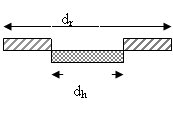A welded pad will be used:

dh = 0.03 m

dr = 2 x dh

= 2x 0.03 = 0.06m

dh = diameter of hole (opening) m

( A factor of 1.5 to 2 is usually used in this equation)

Primary Stress:

There are three types of stress due to the internal pressure.

1. Horizontal (circumferential) stress: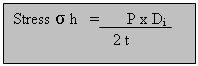Stress h = (3.2x120) /(2x2.7)

= 71.11 N/mm2

P= Internal pressure. N/mm2

Di= Internal diameter.(mm)

t = shell (tube) thickness. (mm)

1. Longitudinal stress: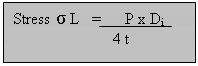Stress L = (3.2x120) / (4x2.7)

= 35.5 N/mm2

P= Internal pressure. N/mm2

Di= Internal diameter.(mm)

t = shell (tube) thickness. (mm)

1. Compressive stress:

For stainless steel E= 200,000 N/mm2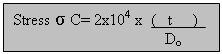Stress C = 2x104 x(2.7/122.7)

= 440 N/mm2

Stress C= critical bulking stress

t = thickness

Do = outside diameter.

The maximum compressive stress should not exceed 440 N/mm2 in the vessel or the vessel may fail by elastic instability.

Ø The vessel shell.

Ø The vessel filling ( e.g. nozzles).

Ø Internal fittings.

Ø External fittings.

Ø Insulations.

Wv = 240 x Cv x Dm (Hv + 0.8 Dm) t

Wv = Total weight of shell, excluding internal fittings.

Cv = a factor to account for the weight of nozzles, internal supports...etc.

( a factor of 1.08 is used with only few internal fittings)

Hv = length of cylindrical section.(m)

Dm= mean diameter of vessel ( Di + t) in (m).

t= shell thickness (mm).

Wv = 240 x 1.08 x 0.1277 (9 + 0.8 x 0.1227 ) x 2.7

= 813 N

Sites in the United Kingdom do not carry out seismic stress analysis in preliminary design of vessels. Since this mechanical design is for a plant in the U.K the seismic stress analysis will neglected. (page 837)

Fs = ae (w/g)

g=the acceleration due to gravity.

ae=the acceleration of the vessel due to earthquake.

W= the weight of the vessel.

1. Dead weight of the vessel.

W= Wv ( = 781 kN) + catalyst weight

Catalyst used= Nickel

Density = 43.5kg.m3

Mass = 43.5x 0.407 = 17.7m3

Weight = mass x g

= 173.5 N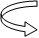Fw = wind pressure x Main diameter

Fw = 1280 N/mm2 x 0.12

Fw = 153.6 N/m

1. Bending moment:

Mx =(Fw/Di) x L2

Mx = (153.6 / 0.12) x 92

Mx = 103680 N/m

§ Analysis Stress:

Ø Pressure Stress

Stress σ L = 35.5 N/mm2

Stress σ h = 71. N/mm2

Stress σ w = Wv /( 3.14 x (Di +t) x t

Stress w = 1044.5 x (3.14 x (120 + 2.7 ) 2.7

Stress w = 1.0 N/mm2 (compressive)

Ø Bending stress:

Do = 122.7 mm (outside diameter)

Iv = (3.14/ 64) x ( Do4 – Di4 )

Iv = the second moment of area of the vessel about the plane of bending.

Iv = (3.14 / 64) x ( 122.7x104 - 120x104)

Iv = 9.4x105

Stress σ b = +/- (M/Iv) x ((Di/2)+t)

Bending stress=

Bending stress=+/- (103680 /9.4x105) x ((120/2)+ 2.7)

Bending stress= 69.15 N/mm2

σ

Ø The resultant longitudinal stress:

Stress σ z = stress l + stress w +/- stress b

(Stress σ w is compressive therefore –ve)

Stress σ z = 35.5 – 1 + 69.15 = 33.65 N/mm2

Stress σ z = 35.5 - 1 - 69.15 = -34.65 N/mm2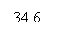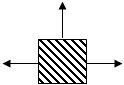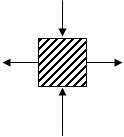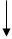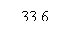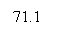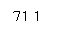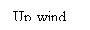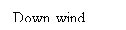The great difference between the principal stresses will be on the down wind side:

(71.1 – (-34.6)) = 107.5 N/mm2

The vessel will stand the internal stress since the difference between the principal stresses is below the design stress.

Support:

§ type of support: Bracket support (Single plate)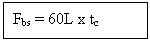1) for the back row tubes :

Fbs = 60 x 150 x 20 =18x 104 N

2)For the front row tubes:

Fbs = 60 x 250 x20 = 30x104 N

L= length of bracket support (mm)

T = thickness of support (mm)

Reference:

Chemical Engineering design, Coulson and Richardson, vol.6, 3rd Edition, section 13.

Vessel Data Sheet

Description (Furnace):Primary Reformer

Type of vessel

Reactor Tubes

No of tubes Required:

614

Capacity

0.105 m3

Contents

Methane and Steam

Premixed

Catalyst

Nickel catalyst

Density

53.5 kg/m3

Diameter

0.12

m

Length

m

Max working Pressure

38

bar

Design Pressure

bar

Max working Temperature

1200

K

Design Temperature

1073

K

Estimation of Temp inducing creep

1315

oC

Materials

Stainless Steel 314

Joint Factor

0.70

Corrosion Allowance

1 mm

Thickness

2.7 mm

End Type

Thickness

2.9 mm

Joint Factor

0.70

End Type

Thickness

2.9 mm

Joint Factor

0.70

Type of support

Brackets

Thickness

20 mm

Material

Steel

6.65 kN/m

None

1044 N

Weight of catalyst

170 N

Bending Stress

15.7 N/mm2

0.42 N/mm2

Max design load of bracket support

372 k/N

Project title: Synthesis of Ammonia from Natural Gas

Company : Zeta Process Engineering

Designed By:

Course: Beng Chemical Engineering

Talal Omar

Project: Design Project

Supervisor

Dr S.Larkai

London South Bank University

Approved:

Dr R.Best

Assignment Help | Mechanical Engineering Assignment Help | Mechanical Engineering Homework Help | Online Tutoring